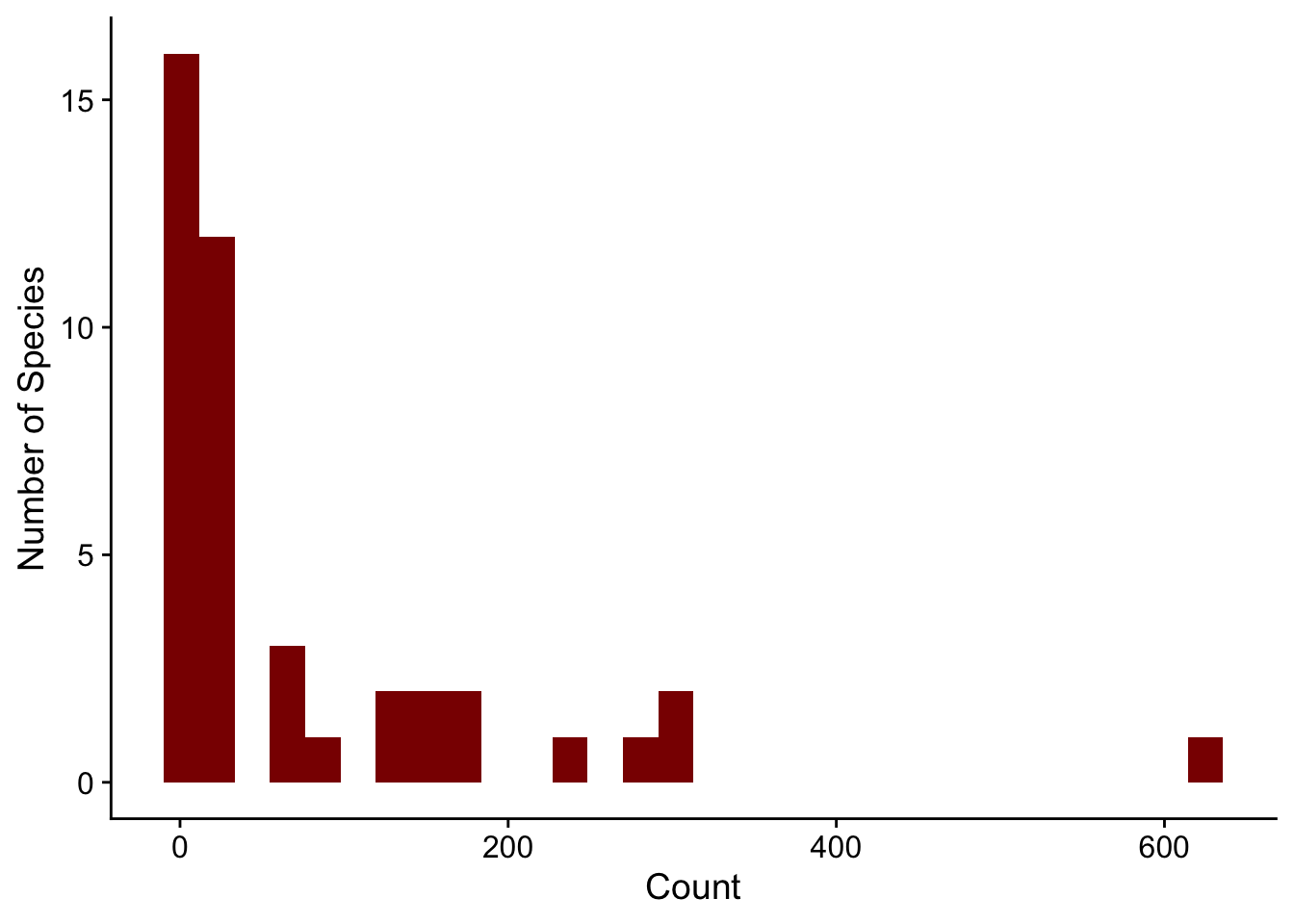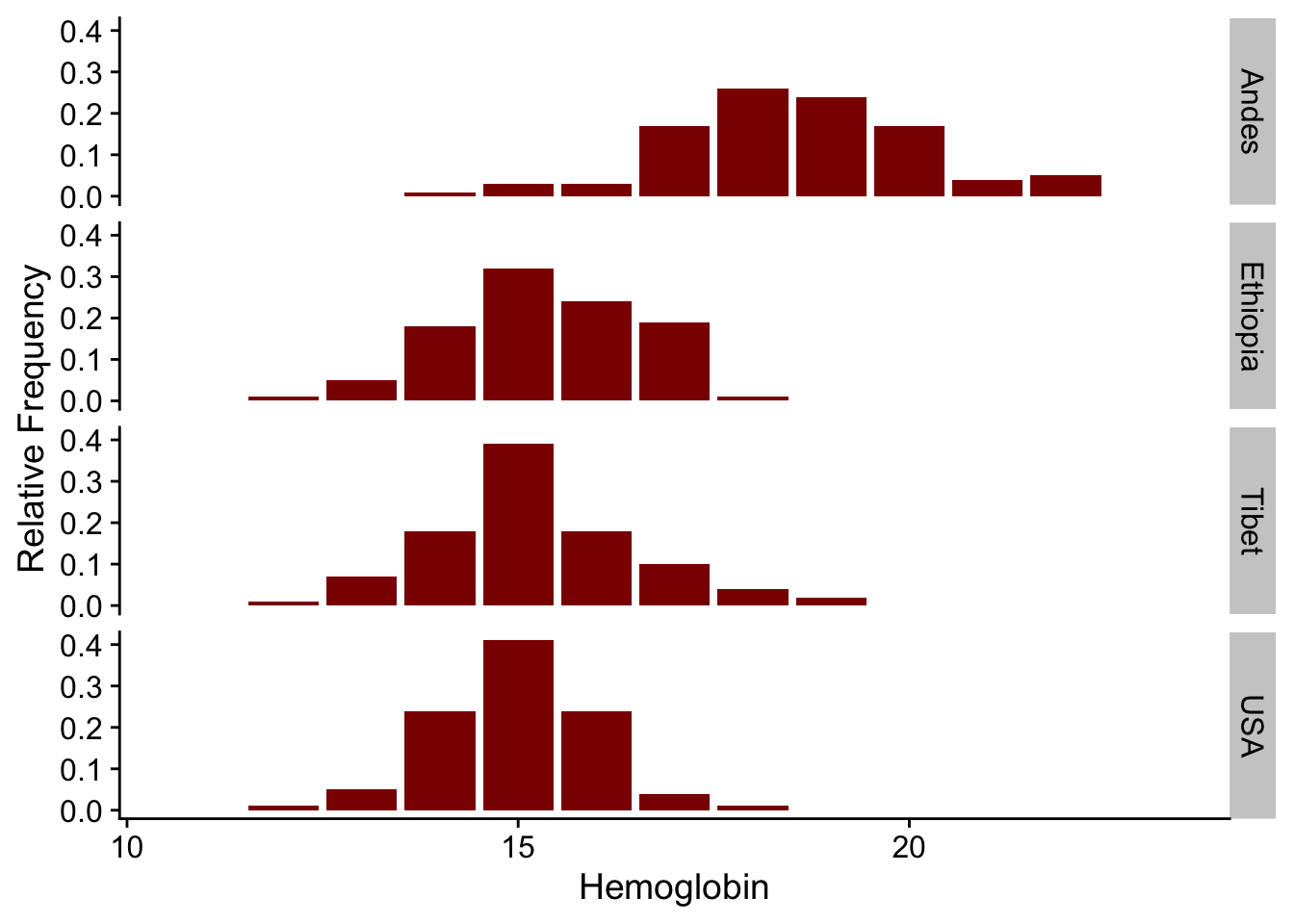# Software

Code for most lab projects is available either on the lab GitHub page or my personal GitHub page.

## `abd`: Analysis of Biological Data

Collaborating with Randall Pruim (Calvin College), I co-developed an R package (`abd`) to accompany the first edition of The Analysis of Biological Data by Michael Whitlock and Dolph Schluter. This is an excellent textbook (now in its second edition, still remarkably inexpensive) for teaching advanced undergraduate and introductory graduate statistics. The new edition of this book has associated R code, mostly making this package redundant. But I still like having the datasets from the book handy. I use them for teaching and demos really often, like the distribution of counts of desert birds or hemoglobin levels in different populations.

``````library(ggplot2)
library(abd)
ggplot(DesertBirds, aes(count)) +
geom_histogram(bins = 30, fill = "darkred") +
labs(x = "Count", y = "Number of Species") +
cowplot::theme_cowplot()````````````library(ggplot2)
library(abd)
ggplot(Hemoglobin, aes(x = hemoglobin, y = relative.frequency)) +
geom_bar(stat = "identity", fill = "darkred") +
labs(x = "Hemoglobin", y = "Relative Frequency") +
facet_grid(group ~ .) +
cowplot::theme_cowplot()``````### Installation

The package can be installed via CRAN

``install.packages("abd")``

or directly from GitHub:

``remotes::install_github("Middleton-Lab/abd")``

We have had a lot of dependency creep over the years, so the number of packages that get installed alongside `abd` can be quite large.

## `jnt`: Johnson-Neyman Technique

The Johnson-Neyman technique is used to determine the region of an analysis of covariance where the slopes are not significantly between the covariate. This allows the determination of bounds of a region of “equal” slope despite the presence of a “significant” slope (by whatever definition of “significant” you choose).

We used this approach in Lavin SR, Karasov WH, Ives AR, Middleton KM, Garland T Jr (2008) Morphometrics of the avian small intestine compared with that of nonflying mammals: A phylogenetic approach. Physiol Biochem Zool 81: 526-550.

### Demo

``library(jnt)``
``Loading required package: smatr``
``````set.seed(1234)

n <- 50

x1 <- rnorm(n)
y1 <- x1 + rnorm(n, sd = 0.2)

x2 <- rnorm(n)
y2 <- 1.25 * x2 + rnorm(n, sd = 0.2)

df1 <- data.frame(x = x1, y = y1)
df2 <- data.frame(x = x2, y = y2)

(jnt.out <- jnt(df1, df2))``````
``Fitting with OLS``
``Assuming x variable is column 1, and y is column 2.``
``````
Johnson-Neyman Technique

Alpha =  0.05

Data 1:
Slope        0.9853
Intercept    0.02126

Data 2:
Slope        1.182
Intercept    0.01386

Region of non-significant slope difference
Lower: -0.5258
Upper: 0.8701 ``````
``plot(jnt.out)``
```geom_smooth()` using formula 'y ~ x'``### Installation

`jnt` can be installed directly from GitHub:

``remotes::install_github("Middleton-Lab/jnt")``